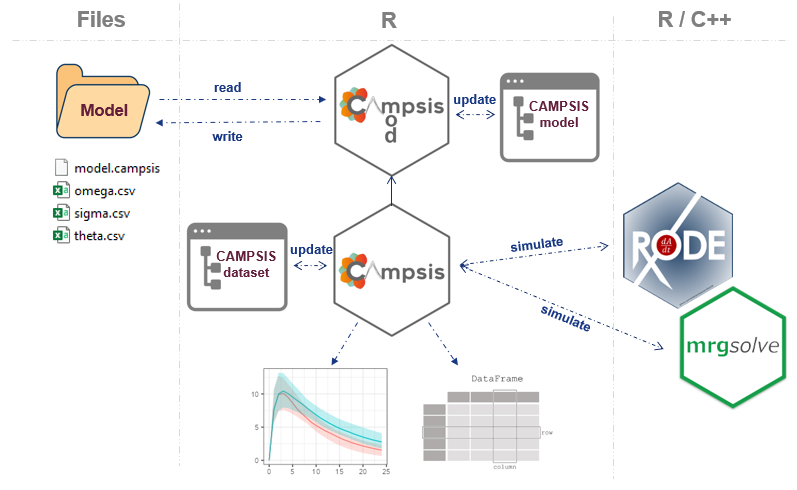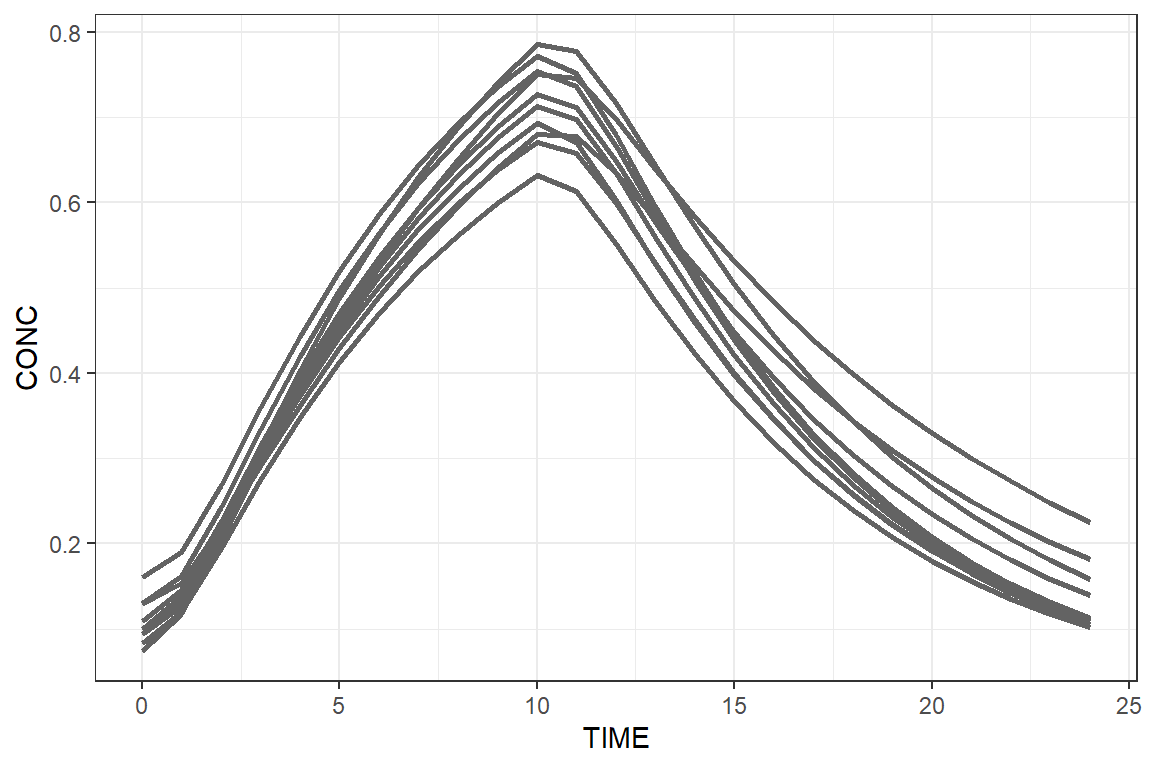## Introduction

CAMPSIS is a open-source PK/PD simulation platform built on top of the open-source simulation packages rxode2, RxODE and mrgsolve. It provides an abstraction layer over the underlying processes of writing a model, assembling a dataset and running, in the background, a simulation with these packages.

CAMPSIS is developed by software engineers working at Calvagone and designed for pharmacometricians who are looking for an easy and intuitive way to run model-based simulations. The packages are developed in R, which makes it possible to integrate your simulation(s) into an R workflow (e.g. standard R script, Rmarkdown, Shiny app, etc.).

## Brief overview

As shown in the schematic below, CAMPSIS comprises two inter-connected R packages: campsismod and campsis. The first package allows the user to assemble, read, write or manipulate a PK/PD model. It also provides useful utilities to export the model to RxODE or mrgsolve. The second package allows the user to assemble the dataset in a very intuitive manner. Once the user’s dataset is ready, the package is in charge of preparing the simulation, calling RxODE or mrgsolve and returning the results, for a given model, dataset and desired simulation settings.## A quick example

Load a 1-compartment model with absorption compartment from the model library.

library(campsis)
model <- model_library\$advan2_trans2

For instance, let’s adapt the population estimate for clearance.

model <- model %>% replace(Theta(name="CL", value=10))

Assume some drug is already present in the central compartment (e.g. 0.1 ng/mL with 20% CV across individuals).

model <- model %>%
add(Omega(name="CENTRAL_INIT", value=20, type="cv%")) %>%
add(InitialCondition(compartment=2, rhs="THETA_CENTRAL_INIT*exp(ETA_CENTRAL_INIT)*V"))

Let’s now create a small dataset of 10 individuals and let’s infuse 100 mg at time 0, for a duration of 10 hours.

dataset <- Dataset(10) %>%
add(Infusion(time=0, amount=100, compartment=1, duration=10)) %>%
add(Observations(0:24))

We can now simulate this very simple model and dataset.

Finally, we can use ggplot2 to plot these results.

library(ggplot2)
ggplot(results, aes(x=TIME, y=CP, group=ID)) +
geom_line(colour="gray39", size=1) + theme_bw()Well done, you just drew the A letter of our CAMPSIS logo.

## Going further

If you haven’t installed CAMPSIS, please visit the installation section.

If you are looking for some more examples, please check out our documentation websites: